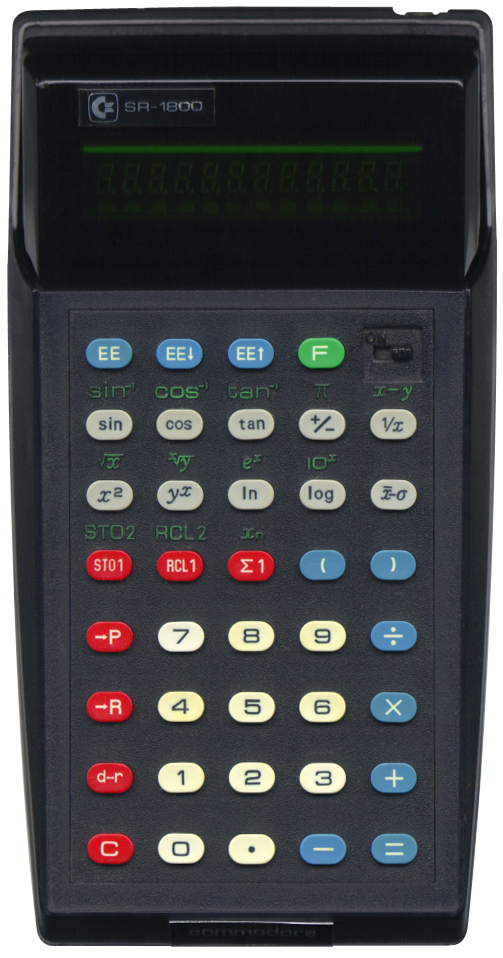# commodore SR‑1800

## Description

 Brand: commodore Model: SR-1800 Type: Scientific calculator Picture:Batteries: AA x 3 Lifetime: Introduced: 1976 Terminated: unknown Notes: Another nice fluorescent display calculator from the mid 1970's made by commodore. This is a scientific calculator with two memories, statistic functions, angle conversions and parenthesis. Some nice and some less nice features that are worth mentioning: The "d-r"-key not only switches between "degrees" and "radians" mode, but also converts the number currently in display from one angle mode to the other. For example, when the calculator is in "degrees" mode, and the number 180 is displayed, pressing the "r"-key will convert the angle to π degrees. When the calculator is in "radians" mode, the rightmost dot on the display is lit. The "→P" and "→R" keys convert between vector modes. The parameters need to be present as "x" and "y", and can be entered using the "x↔y"-key. The results can be read using the same key. The "EE↑"-key and "EE↓"-key shift left and right in scientific notation. However, when the number shifts out of display, the number is truncated. This can not be used to round the number, because no rounding off takes place. The "Σ1"-key is what is nowadays usually denoted by "M+", it adds the number displayed to the first of the two memories. This calculator is particularly inaccurate. When pressing a number and then pressing the "yx"-key, the natural logarithm of y is shown as an intermediate result. This is probably due to the fact that the calculator uses the formula yx = ex⋅ln y.